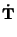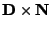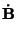## Darboux Vector

The rotation Vector of the Trihedron of a curve with Curvaturewhen a point moves along a curve with unit Speed. It is given by(1)

whereis the Torsion, T the Tangent Vector, and B the Binormal Vector. The Darboux vector field satisfies(2)(3)(4)